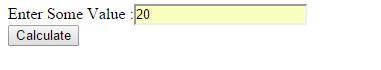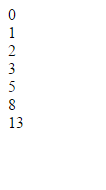## Sunday, 18 January 2015

### Fibonacci Series with JavaScript

This is a simple Java Script code which displays the fibonacci series upto the range given.

First we create a text field to enter some value and a button which on clicked calls the JavaScript function.

The HTML part is ,
```Enter Some Value :<input type = "text" id= "val" ></input></br>
<input type = "button" value ="Calculate"  onclick="fibonacci()">```

then it looks like,JavaScript  - Fibonacci Series

Now when  we enter the value and click the 'Calculate' button the script for calculating fibonacci will run,
```<script type="text/javascript">
function fibonacci(){
var a = 0, b = 1, c = 0;
var res = document.getElementById("val").value;

while (b <= res) {

document.write(c);
document.write("</br>");

c = a + b;
a = b;
b = c;

}
}
</script>```JavaScript  - Fibonacci Series

The function fibonacci()  gets the input from the html by,
`var res = document.getElementById("val").value;`

now we will perform the actual logic for generating finonacci series
```while (b <= res) {

document.write(c);
document.write("</br>");

c = a + b;
a = b;
b = c;

}```JavaScript  - Fibonacci Series

The complete HTML file  code is as below,
```<html>
<body>
Enter Some Value :<input type = "text" id= "val" ></input></br>
<input type = "button" value ="Calculate"  onclick="fibonacci()">
<script type="text/javascript">
function fibonacci(){
var a = 0, b = 1, c = 0;
var res = document.getElementById("val").value;

while (b <= res) {

document.write(c);
document.write("</br>");

c = a + b;
a = b;
b = c;

}
}

</script>
</body>
</html>```

OUTPUT :
Enter Some Value :